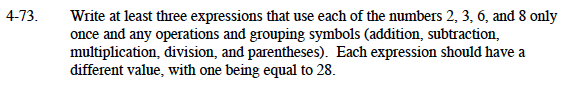### Home > MC1 > Chapter 4 > Lesson 4.2.3 > Problem4-73

4-73.

. Write at least three expressions that use each of the numbers 2, 3, 6, and 8 only once and any operations and grouping symbols (addition, subtraction, multiplication, division, and parentheses). Each expression should have a different value, with one being equal to 28. Homework Help ✎Refer to problem MC1 4-17 for help.

The expression that is equal to 28 follows the following format:
( ? )( ? ) + ? − ? = 28
Each ? represents one of the four given numbers.